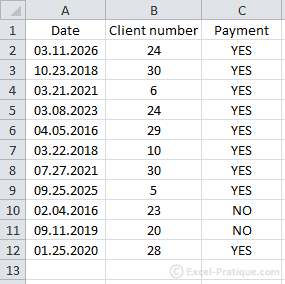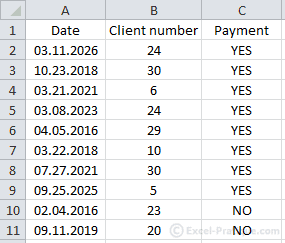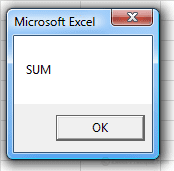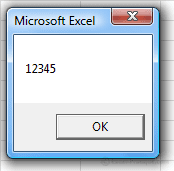# VBA Course: Using Arrays (continued)

## The two dimensional array

To store more than one column of data, we will need another dimension in our array. Here is an example:Storing data in a 2 dimensional array:

`'DeclarationDim array_example(10, 2) '11 x 3 "element" array'Storing values in the arrayFor i = 0 To 10    array_example(i, 0) = Range("A" & i + 2)    array_example(i, 1) = Range("B" & i + 2)    array_example(i, 2) = Range("C" & i + 2)Next`

And here are a few examples of working with these values:

`MsgBox array_example(0, 0) '=> returns: 03.11.2026MsgBox array_example(0, 1) '=> returns: 24MsgBox array_example(9, 2) '=> returns: NOMsgBox array_example(10, 2) '=> returns: YES`

## The dynamic array

Just imagine for a moment that this same data set was going to be updated regularly and therefore we couldn't set fixed values when we declare it ...To find out the row number of the last cell in a series of non-empty cells, or in other words, the last row in our database, we'll use the following formula:

`last_row = Range("A1").End(xlDown).Row`

Excel does not accept variables in declarations.

Instead, declare a dynamic array (using empty parentheses), then define its dimensions using Redim:

`Dim array_example()ReDim array_example(last_row - 2, 2)`

Using the following procedure, you can store all the rows in the data set in your array:

`Sub example()    last_row = Range("A1").End(xlDown).Row 'Last row of the data set    Dim array_example()    ReDim array_example(last_row - 2, 2)        'Storing values in the array    For i = 0 To last_row - 2        array_example(i, 0) = Range("A" & i + 2)        array_example(i, 1) = Range("B" & i + 2)        array_example(i, 2) = Range("C" & i + 2)    NextEnd Sub`

## Ubound

In the preceding example, the last number in our array was last_row - 2:

`For i = 0 To last_row - 2`

Another way to determine the last number in the array would be to use Ubound:

`For i = 0 To UBound(array_example)`

This function returns the highest number in the array for the chosen dimension (the first dimension is the default).

Here are a few examples that will make this clearer:

`Sub example()    Dim array_example(10, 2)        MsgBox UBound(array_example) '=> returns: 10    MsgBox UBound(array_example, 1) '=> returns: 10    MsgBox UBound(array_example, 2) '=> returns: 2End Sub`

## Storing data in a range of array elements

It's possible to populate an array with the data from a range of worksheet cells without even using a loop.

`'DeclarationDim array_example(10, 2) '11 x 3 "element" array'Storing values in the arrayFor i = 0 To 10    array_example(i, 0) = Range("A" & i + 2)    array_example(i, 1) = Range("B" & i + 2)    array_example(i, 2) = Range("C" & i + 2)Next`

The preceding code can effectively be replaced with:

`'DeclarationDim array_example()'Storing values in the arrayarray_example = Range("A2:C12").Value`

But if this second method seems attractive at first, be warned that in many cases it can cost you more time than the first one ...

If you store data in your array in this way, the first number will be 1 rather than 0, which can cause confusion ... Further along in the development process, if you decide to save only data that correspond to certain search criteria in your array (or to carry out an entirely different operation), you will have to entirely rewrite the code using another loop function ...

But this second method is quite useful if you need to store the entire contents of a large data set, because it's faster than a loop (saving about 0.2 seconds for every 15,000 entries).

## Array

But if you need to create an array that has "fixed" contents.

One solution would be to set the contents line by line:

`Dim en(5)en(0) = "IF"en(1) = "VLOOKUP"en(2) = "SUM"en(3) = "COUNT"en(4) = "ISNUMBER"en(5) = "MID"`

Luckily, you can simplify this code by using Array:

`en = Array("IF", "VLOOKUP", "SUM", "COUNT", "ISNUMBER", "MID")`

Here is a demonstration of the use of the Replace function (this will help you understand the example that follows):

`Sub replace_example()    Dim var_translate As String    'A string for this example    var_translate = "Hello World !"        'Replacement of "World" with "you" in the character string    var_translate = Replace(var_translate, "World", "you")    'The string after replacement    MsgBox var_translate '=> returns "Hello you !"End Sub`

Now if we want to replace a series of values with another series, using arrays and the (Array) function will be extremely helpful:

`Sub translate() 'Simplified example of EN-FR translation for formulas    Dim var_translate As String    'A string for this example    var_translate = "Formula to translate: SUM(IF(ISNUMBER(A1:E1),A1:E1,0))"        'The two series of values    en = Array("IF", "VLOOKUP", "SUM", "COUNT", "ISNUMBER", "MID")    fr = Array("SI", "RECHERCHEV", "SOMME", "NB", "ESTNUM", "STXT")        'Replacing "SI" with "IF", and "RECHERVEV" with "VLOOKUP", etc.    For i = 0 To UBound(en)        var_translate = Replace(var_translate, en(i), fr(i))    Next    'The string after the replacements    MsgBox var_translate '=> returns "Formula to translate: SOMME(SI(ESTNUM(A1:E1),A1:E1,0))"End Sub`

## Split

The Split function allows us to convert a character string into an array.

To convert the string into an array, do the following:

`variable = "IF/VLOOKUP/SUM/COUNT/ISNUMBER/MID"`

Use the Split function and define the separator:

`en = Split(variable, "/")`

The array en will return the following values:

`MsgBox en(0) '=> returns: IFMsgBox en(1) '=> returns: VLOOKUPMsgBox en(2) '=> returns: SUMMsgBox en(3) '=> returns: COUNTMsgBox en(4) '=> returns: ISNUMBERMsgBox en(5) '=> returns: MID`

The following 3 arrays will also return the same values:

`en = Array("IF", "VLOOKUP", "SUM", "COUNT", "ISNUMBER", "MID")en = Split("IF,VLOOKUP,SUM,COUNT,ISNUMBER,MID", ",")en = Split("IF VLOOKUP SUM COUNT ISNUMBER MID", " ")`

The following example returns the 3rd value in the string:

`MsgBox Split("IF,VLOOKUP,SUM,COUNT,ISNUMBER,MID", ",")(2) '=> returns: SUM`The opposite of the Split function is Join.

This function assembles the values of an array into a string.

`MsgBox Join(Array(1, 2, 3, 4, 5), "") '=> returns: 12345`